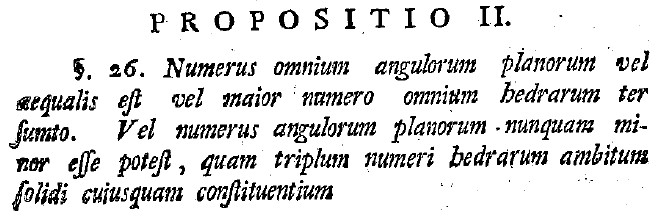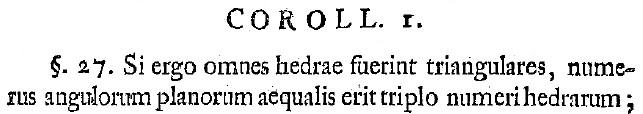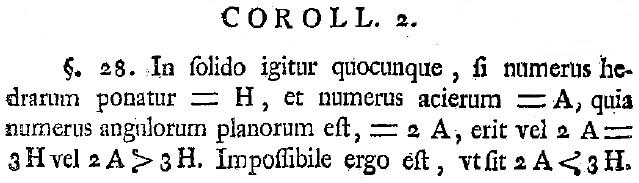# Investigating Euler's Polyhedral Formula Using Original Sources - Euler's Proposition 2

Author(s):

As we progress through the paper, our vocabulary becomes larger and the propositions become easier to comprehend. Euler's second Proposition (paragraph 26) is:

Numerus omnium angulorum planorum vel aequalis est vel maior numero omnium hedrarum ter sumto.
Vel numerus angulorum planorum nunquam minor esse potest, quam triplum numeri hedrarum ambitum solidi cuiusquam.Figure 6. Euler's Proposition 2.

Many of these words are familiar by now; we discuss those which are new. The word vel means "or"; the construction "vel . . . vel" means "either . . . or". We may guess that aequalis means "equal(s)". The word maior looks unfamiliar, but we may try swapping the "i" with a "j" as described earlier. Major we will translate as "greater". The word ter is the root of words such as "tertiary"; we will thus translate ter as "three". Sumto appears to have sum as a root; this might mean "sum" or "addition". In parallel with our translation of major above, we will translate minor as "lesser". Using our Latin-to-English dictionary, we guess that esse will mean "is", potest will mean "possible", quam could mean "who", "what", or "which", and nunquam could mean "at no time", "never", or "in no circumstances". It is a reasonable guess that triplum means "triple". And so our rough first translation becomes:

Number all plane angles either equal is or greater number all faces three sum.
Or number plane angles never lesser is possible, which triple number faces around solid any.

Gently rearranging words and using articles, our attempt at translation yields:

The number of all the plane angles is either equal to or greater than thrice (three times) the number that is the sum of all the faces.
The number of plane angles can never possibly be less than triple the number of faces around any solid.

Mathematically speaking, this proposition states (in modern notation) that:

Either $P = 3F$ or $P > 3F$.
It is never the case that $P < 3F$.

This is slightly more complicated than the last proposition. Students could find the proof using guided discovery or by translating Euler's line of reasoning -- whatever method works best for the course and students at hand. Again, it might be helpful for students to see examples of solids for which the equality holds and for which the inequality holds. Teachers could prepare a batch of examples for use in the classroom, or see what Euler has to say in Corollary 1 to this proposition:

Si ergo omnes hedrae fuerint triangulares, numerus angulorum planorum aequalis erit triplo numeri hedrarum. . . .Figure 7. Euler's Corollary 1 to Proposition 2.

The only new word here is ergo, which we may recognize as part of the famous quote "cogito ergo sum"—"I think, therefore I am". Thus we translate ergo as "therefore", and our rough first translation is:

If therefore all faces is triangle(s), number plane angles equal is triple number faces. . . .

A slight revision would yield:

Therefore, if all faces are triangles, the number of plane angles is equal to triple the number of faces. . . .

Again, the word "therefore" arises because a corollary follows from the proposition appearing before it. Euler has given us an example of when equality holds in Proposition 2. Why not ask students to check it for the tetrahedron, octahedron, and dodecahedron?

Let us examine the second corollary of Proposition 2.

In solido igitur quocunque, fi numeris hedrarum ponatur = H, et numerus acierum = A,
quia numeris angulorum planorum =
2A, erit vel 2A = 3H vel 2A > 3H.
Impossibile ergo est, ut sit
2A < 3H.Figure 8. Euler's Corollary 2 to Proposition 2.

The further we progress, the easier the translations become! New words appearing here include ponatur, which could mean "put" or "set" (we will use the latter), quia, which we look up and find means "because", and ut, which we will translate as "while". The rough translation is:

In solid therefore whatever, if number faces set = $H$, and number edges = $A$,
because number plane angles = $2A$, is either $2A = 3H$ or $2A > 3H$.
Impossible therefore is, while is $2A < 3H$.

Cleaning up the translation (and using modern notation), we have:

Therefore, in whatever solid, if the number of faces is set equal to $F$, and the number of edges equals $E$,
because the number of plane angles equals $2E$, it is either [the case that] $2E = 3F$ or $2E > 3F$.
While therefore it is impossible that $2E < 3F$.

In short:

Either $2E = 3F$ or $2E > 3F$; It is never the case that $2E < 3F$.

The proof of this is easy: we are doing a simple substitution, combining Proposition 1 and Proposition 2. This would be such a small step that most authors would omit it, much less set it apart as a corollary by itself. Euler, however, is not most authors. He sets a good pace for students of mathematics, from any century, seeing this for the first time.

Proposition 3 is similar to Proposition 2, and therefore we omit a discussion of it here, although it is still important and should be discussed as it will be important later. Proposition 3, in modern notation, states that

Either $P = 3V$ or $P > 3V$; It is never the case that $P < 3V$.

The second corollary to this proposition states that:

Either $2E = 3V$ or $2E > 3V$; It is never the case that $2E < 3V$.

Lee Stemkoski (Adelphi University), "Investigating Euler's Polyhedral Formula Using Original Sources - Euler's Proposition 2," Convergence (April 2010), DOI:10.4169/loci003297

## Dummy View - NOT TO BE DELETED

••••